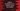# Python program to exchange two numbers without using a third number## Python program to exchange the values of two numbers without using a third number :

In this example, we will learn how to exchange two numbers without using any extra variable. We all know to exchange two numbers using a temporary variable. Exchanging two numbers using a third temporary variable is easy. The working process is the same in all programming languages.

Suppose variable first and second are holding the first and the second number. We want to exchange the values i.e. the first variable will hold the second number and the second variable will hold the first number.

To solve this problem using a third variable, create one temporary third variable first. Assign the value of the first variable to the third variable, assign the value of the second variable to the first variable and then assign the value of the third variable to the second variable. It will exchange both numbers or the numbers holding by the first and the second variable will be exchanged.

But how to solve it without using a third variable? Let’s have a look :

### The algorithm to exchange two numbers without using the third number :

We can easily exchange two variables using basic addition and subtraction. The following steps will show you how to do that :

1. Get inputs of both numbers from the user. Store them in two different variables.
2. Add both numbers and store it in the first number variable.
3. Subtract the second number from the first number and store it in the second number variable. This will subtract the second number from the sum of both numbers. The result will be the original first number. We are storing this value in the second variable, i.e. we are storing the first number in the second variable in this step.
4. Subtract again the_ second number from the first number _and store it in the first number variable. The second variable is changed to the first number in the third step above. So, we are subtracting the first number from the sum. The result is the original second number. In this step, we are storing the second number in the first variable.
5. Now, both numbers are exchanged i.e. first number variable holds the second number and the second number variable holds the first number.

### Python program :

``````#1
first = int(input("Enter the value of the first number : "))
second = int(input("Enter the value of the second number : "))
#2
first = first + second
#3
second = first - second
#4
first = first - second
#5
print("After exchange, First number is : ",first," Second number is : ",second)``````

The source code is available here.

### Explanation :

1. The commented numbers in the above program denote the step number below : Ask the user to enter the first and second number and store it in the variable first and second. For example, the user entered 1 for first and 2 for second.
2. Add both first and second and assign the value to first. Now, first become 1 + 2 = 3
3. Subtract second from first and store it in second. Now second is 3 – 2 = 1 i.e. the initial value of first.
4. Subtract second from first and store it in first. first become 3 – 1 = 2 i.e. the initial value of the second.
5. Print out the final values of first and second.

## Conclusion :

You can see that we can easily exchange two numbers without using a third variable in python. The same algorithm can be used with any other programming language. Try to run the example above and drop one comment below if you have any queries.